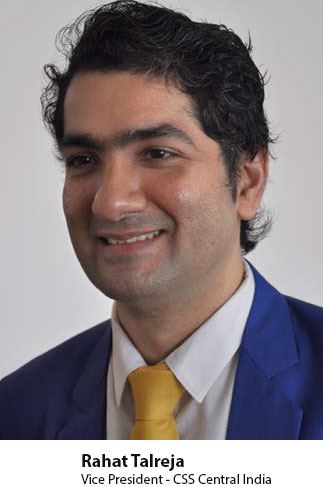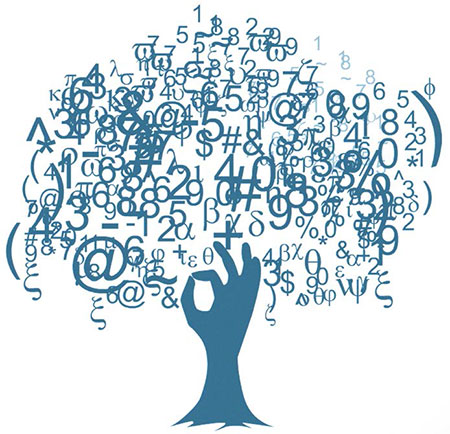## Taste of IndiaIn this series, I would like to bring out the real taste of India like Vedic maths, Hemachandra numbers etc which are fascinating concepts and also explain many complex realities of the universe in simple terms. Today we start with Vedic maths.

Vedic Maths

Any number multiplied by 11:

32 times 11 :

Split 3 and 2

3 2

Add 3 and 2 = 5 and put it in between

Similarly,

45 times 11 = 495

75 times 11 = 7125 —> 1 gets carried over and it becomes 825

Base method ( can be used for any numbers close to bases of 10,100,1000 etc )

99 times 97

100 is the base

99 is less than 100 by how much = 01

97 is less than 100 by how much = 03

Now cross subtract : 99 less 3 = 96

Or

97 less 1 = 96

Each way the answer is same so 96 is the first part.

And now multiply the difference 01 times 03 = 03.

Do it with 98 times 97 , answer is 9506.

996 times 997Now take 1000 as the base

996 is less than 1000 by how much = 004

997 is less than 1000 by how much = 003

Now cross subtract : 996 less 003 = 993

Or

997 less 004 = 993

Each way the answer is same so 993 is the first part.

and now multiple the difference 004 times 003 = 012.

14 times 12

Base is 10

14 is 4 more than 10

12 is 2 more than 10

First part is 16.

Multiply difference 4 times 2 = 8

Mental squaring

101 square = 101 times 101

By the same method above, answer is 10201.

102 square = 102 times 102

By the same method, answer is 10404

105 square : 11025

106 square : 11236

Vertically and crosswise sutra:

31 times 12

31

X12

Formula:

a) :: —> multiply last digit of each row for last number

b) :: —> cross multiply 1st digit of 1st line with 2nd digit of second line

cross multiply 2nd digit of 1st line with 1st digit of second line

Add the two for middle number

c) :: —> multiply first digit of each row for 1st number

a. 1 times 2 = 2 – last number

b. 3 times 2 = 6 + 1 times 1 = 1 add the two = 7 – middle number

c. 3 times 1 = 3 – first number

Now 12 times 34

12

X 34

a. 2 times 4 = 8 – last number

b. 1 times 4 = 4 and 2 times 3 = 6.
4+ 6 = 10 so carry over 1 – middle number is 0.

c. 1 times 3 is 3 + carry over 1 = 4 – first number

So the few methods with a few examples of Vedic maths should help you become smarter and not depend always on your smart phone.

Keep massaging your brain and relish the taste of India

Coming up soon with Hemachandra numbers in the next series !